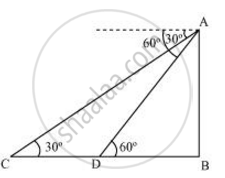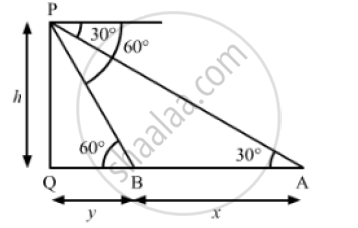# A Straight Highway Leads to the Foot of a Tower. a Man Standing at the Top of Tower Observes a Car as an Angle of Depression of 30°, Find the Time Taken by Car to Reach Foot of Tower from this Point. - Mathematics

A straight highway leads to the foot of a tower. A man standing at the top of the tower observes a car as an angle of depression of 30°, which is approaching the foot of the tower with a uniform speed. Six seconds later, the angle of depression of the car is found to be 60°. Find the time taken by the car to reach the foot of the tower from this point.

#### Solution 1Let AB be the tower.

Initial position of the car is C, which changes to D after six seconds.

AB/DB = tan 60º

(AB)/(DB) =sqrt3

DB = (AB)/sqrt3

In ΔABC,

AB/BC = tan 30º

(AB)/(BD + DC) = 1/sqrt3

ABsqrt3 =  BD + DC

ABsqrt3 = (AB)/sqrt3 + DC

DC = ABsqrt3 - (AB)/sqrt3 = AB(sqrt3 - 1/sqrt3)

= (2AB)/sqrt3

Time taken by the car to travel distance DC ("i.e" "2AB"/sqrt3) = 6 second

Time taken by the car to travel distance DB ("i.e" (AB)/sqrt3) = 6/((2AB)/sqrt3)xx(AB)/sqrt3

= 6/2 = 3 seconds

#### Solution 2Let PQ be the tower.
We have,
∠PBQ = 60° and ∠PAQ = 30°
Let PQ = h, AB = x and BQ = y
In ΔAPQ,

tan 30° = (PQ)/(AQ)

⇒ 1/ sqrt(3) = h/(x+y)

⇒ x+y = h sqrt(3)                  ..................(1)

Also, in   ΔBPQ,

tan 60° = ( PQ)/(BQ)

⇒ sqrt(3) = h/y

⇒ h = y sqrt(3)                    ...............(2)

Substituting  h = y sqrt(3) in (i), we get

x +y = sqrt(3)  (ysqrt(3))

⇒  x + y = 3y

⇒ 3y - y = x

⇒ 2y = x

⇒  y = x/2

"As, speed of the car from "A to B = (AB) /6 = x/6  units/ sec

So, the time taken to reach the foot of the tower i.e. Q from B (BQ)/(speed)
=y/((x/6))

=((x/2))/((x/6))

=6/2

= 3 sec

So, the time taken to reach the foot of the tower from the given point is 3 seconds.

Concept: Heights and Distances
Is there an error in this question or solution?

#### APPEARS IN

NCERT Class 10 Maths
Chapter 9 Some Applications of Trigonometry
Exercise 9.1 | Q 15 | Page 205
RS Aggarwal Secondary School Class 10 Maths
Chapter 14 Height and Distance
Exercises | Q 14

Share